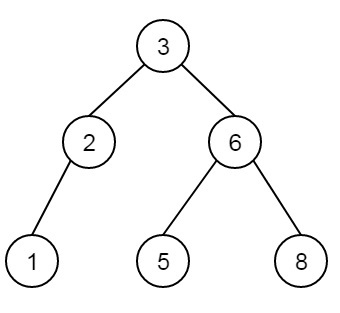# Find the node with minimum value in a Binary Search Tree in C++

C++Server Side ProgrammingProgramming

Suppose we have one binary search tree. We have to find the minimum element in binary search tree. So if the BST is like below −The minimum element will be 1.

As we know that the left subtree always holds the smaller elements. So if we traverse through the left subtree again and again until left is null, we can find the smallest element.

## Example

Live Demo

#include<iostream>
using namespace std;
class node{
public:
node *left;
int val;
node *right;
};
node *bst = NULL;
node *getNode(){
node *newNode;
newNode = new node;
return newNode;
}
void insert(node **root, int key){
node *newNode;
newNode = getNode();
newNode->val = key; newNode->left = NULL; newNode->right = NULL;
if(*root == NULL){
*root = newNode;
return;
} else {
if(key < (*root)->val)
insert(&((*root)->left), key);
else
insert(&((*root)->right), key);
}
}
int minElement(){
node* current = bst;
while (current->left != NULL) {
current = current->left;
}
return(current->val);
}
main(){
int item[] = {3, 2, 1, 6, 5, 8};
int n = sizeof(item)/sizeof(item);
int i;
for(i = 0; i<8; i++){
insert(&bst, item[i]);
}
cout << "Minimum element is: " << minElement();
}

## Output

Minimum element is: 1